# Negative and fractional exponents

By Martin McBride, 2023-02-10
Tags: exponential exponent power root
Categories: exponentialsWe have seen how to calculate the exponential function ax for any integer value of x of 2 or greater.

Now we will extend this to cover:

• Rules for one and zero powers.
• Negative exponent rule.
• Fractional exponent rule.

## Assumptions

We will make some further assumptions:

1. All values are real. It is possible to calculate exponentials involving complex numbers, we won't cover it here.
2. The base is not negative. This is because exponential equations for negative bases can yield complex number results for certain values of x.
3. The base is not zero. This is because exponential equations for base zero are undefined results for certain values of x.

This means that we will assume the base is positive and the exponent is any real number.

## Rules for one and zero powers.

The exponentials a2, a3. a4 form a series where each item is equal to the previous term multiplied by a. Every time we multiply by a, the power increases by 1.

We can also generate the series in reverse order like this: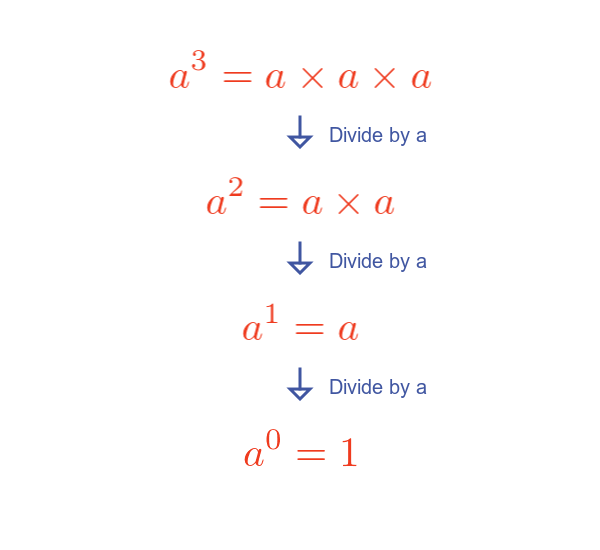Starting from a3, each time we divide the item by a, the exponent decreases by 1.

This allows us to find that the value of:

• $a^1$. This is a. This makes sense because one multiplied a once is just a.
• $a^0$. Again, by the original definition, this is one multiplied by a zero times, which is one.

## Special bases 1 and 0

Powers of one, such as 1, 1 × 1, and 1 × 1 × 1, are all equal to 1. We can say that 1 raised to any power is 1.

Similarly, 0, 0 × 0, and 0 × 0 × 0 are all equal to 0. So 0 raised to any power is 0.

But there is a special case. Previously we saw that any number raised to the power 0 is equal to 1. But we also know that 0 raised to any (non-negative) power is 0. So what is 00?

We say that this value is undefined. This is similar to the case of 0 divided by 0, which is also undefined.

As we said earlier, we are only considering positive bases in this article, and since 0 is not positive we do not need to worry about the special case.

## Negative exponent rule.

Suppose we carry on with the previous technique and keep dividing by a and reducing the exponent by 1: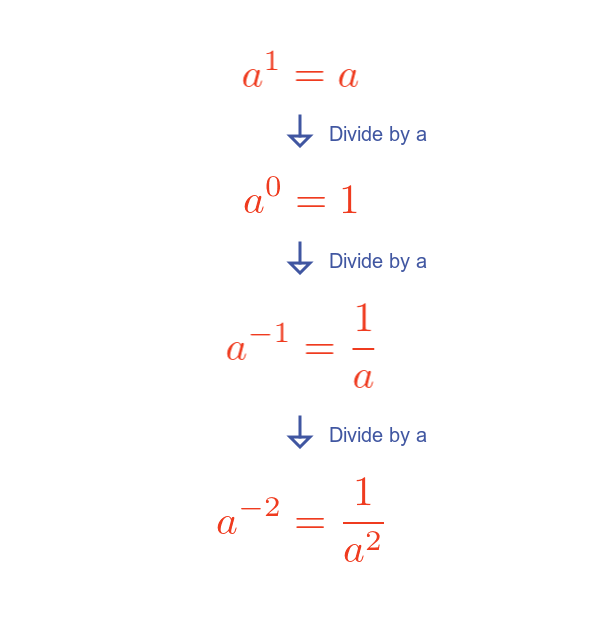This tells us that a-1 is equal to the reciprocal of a.

If we use the definition we expect that a-1 is one multiplied by a minus one times. What does that mean? Well if we interpret that as meaning we do the opposite of multiplying, one time, it means that the result should be one divided by a. Which is exactly what it is.

In general a-p is the reciprocal of ap.

## Fractional exponent rule.

Can we raise a to the power one-half? And what would that mean? Here is an illustration: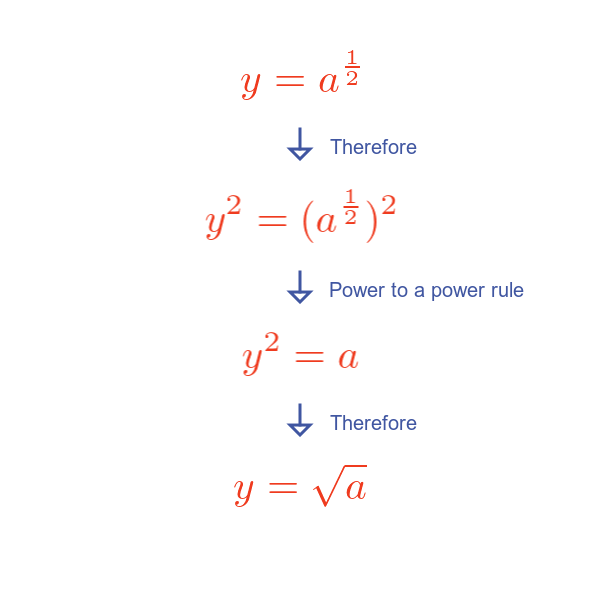We set y equal to a to the power one half (whatever that might be).

Using the power to a power rule, a raised to the power 1/2, then raised the power 2, is just equal to a.

This means that y2 to equal a, making y equal to the square root of a.

Incidentally, this is an example of why we are only considering positive bases. If a was -1, we would need to calculate the square root of -1, which has no real number value.

But the same logic, a to the power one-third is the cube root of a: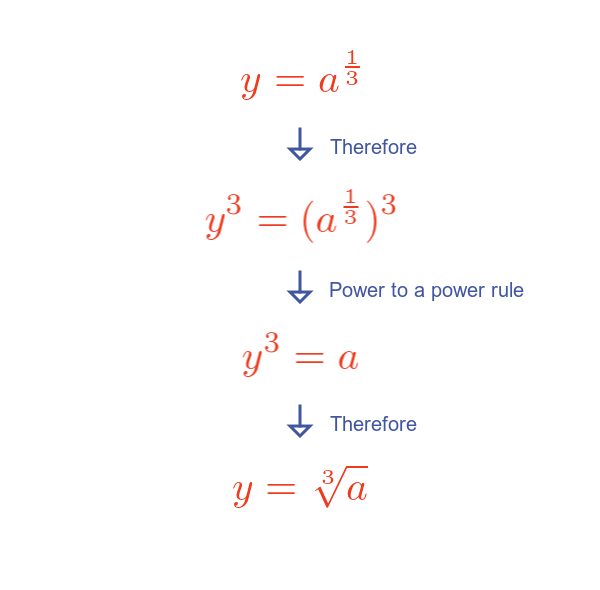This can be extended to include any rational number as a power: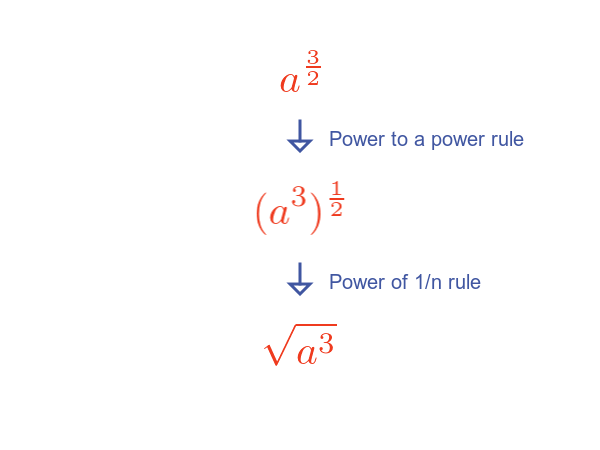Here we use the fact that raising a to the power of 3/2 is equivalent to raising a to the power of 3 followed by raising it to the power of 1/2. In that way, any rational power can be calculated by raising a to an integer power and then taking an integer root.

The article Exponential function for irrational values of x shows this can be extended to cover irrational valued powers.